# Math in Focus Grade 5 Chapter 6 Practice 3 Answer Key Finding the Area of a Triangle

Practice the problems of Math in Focus Grade 5 Workbook Answer Key Chapter 6 Practice 3 Finding the Area of a Triangle to score better marks in the exam.

## Math in Focus Grade 5 Chapter 6 Practice 3 Answer Key Finding the Area of a Triangle

Example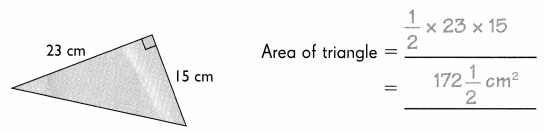Question 1.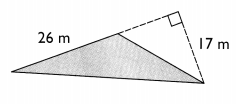Area of a triangle = _____
= ________
Height = 26 m
Base = 17m
Area of the triangle = 1/2 × b × h
= 1/2 × 17 × 26
= 221 m2

Question 2.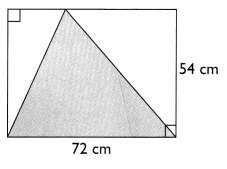Area of a triangle = _____
= ________
From the above figure Base of the triangle is 72 cm. Height of the triangle is 54 cm.
Area of the triangle = 1/2 × b × h
= 1/2 × 72 × 54
= 1,944 cm2

Question 3.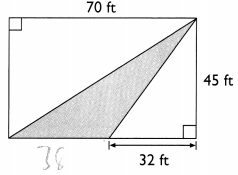Area of triangle = ____
= _______
From the above figure Base of the triangle is 38 ft. Height of the triangle is 45 ft.
Area of the triangle = 1/2 × b × h
= 1/2 × 38 × 45
= 855 ft2

Find the area of each shaded triangle.

Example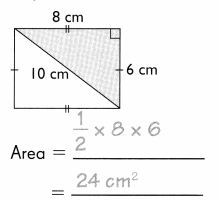Question 4.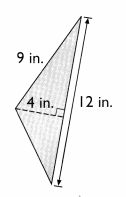Area = ____
= ______
From the above figure Base of the triangle is 12 in. Height of the triangle is 4 in.
Area of the triangle = 1/2 × b × h
= 1/2 × 12 × 4
= 24 in2

Question 5.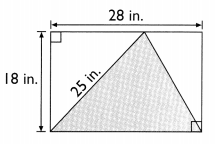Area = _____
= ______
From the above figure Base of the triangle is 28 in. Height of the triangle is 18 in.
Area of the triangle = 1/2 × b × h
= 1/2 × 28 × 18
= 252 in2

Question 6.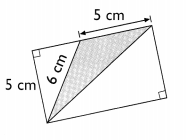Area = _____
= ______
From the above figure Base of the triangle is 5 cm. Height of the triangle is 5 cm.
Area of the triangle = 1/2 × b × h
= 1/2 × 5 × 5
= 25/2 cm2

Question 7.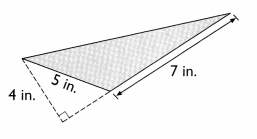Area = ____
= ______
From the above figure Base of the triangle is 7 in. Height of the triangle is 4 in.
Area of the triangle = 1/2 × b × h
= 1/2 × 7 × 4
= 14 in2

Question 8.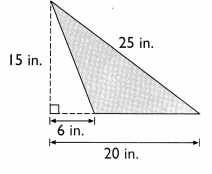Area = _____
= _______